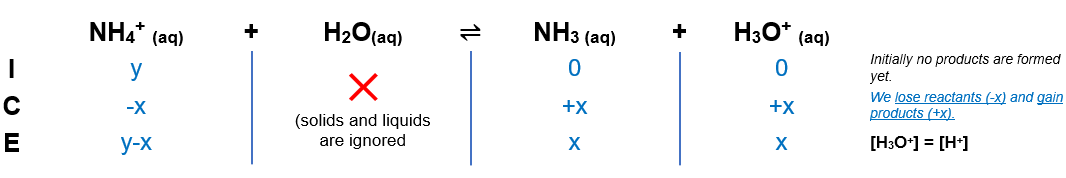# Problem: Ammonium (NH4+) is a weak acid with a pK a of 9.26. The dissociation is:NH4+ ⇌ NH3 + H+Calculate the mass of ammonium added to 1 L of water in order to obtain a pH of 4 at equilibrium.

###### FREE Expert Solution

(1) Find the Ka and H+ from pKa and pH

Ka = 10-pKa = 10-9.26 = 5.50x10-10

(2) Set up ICE table and find initial NH4+ where x = 0.0001(3) Find y where x = 0.0001.

94% (317 ratings)###### Problem Details

Ammonium (NH4+) is a weak acid with a pK a of 9.26. The dissociation is:

NH4+ ⇌ NH3 + H+

Calculate the mass of ammonium added to 1 L of water in order to obtain a pH of 4 at equilibrium.